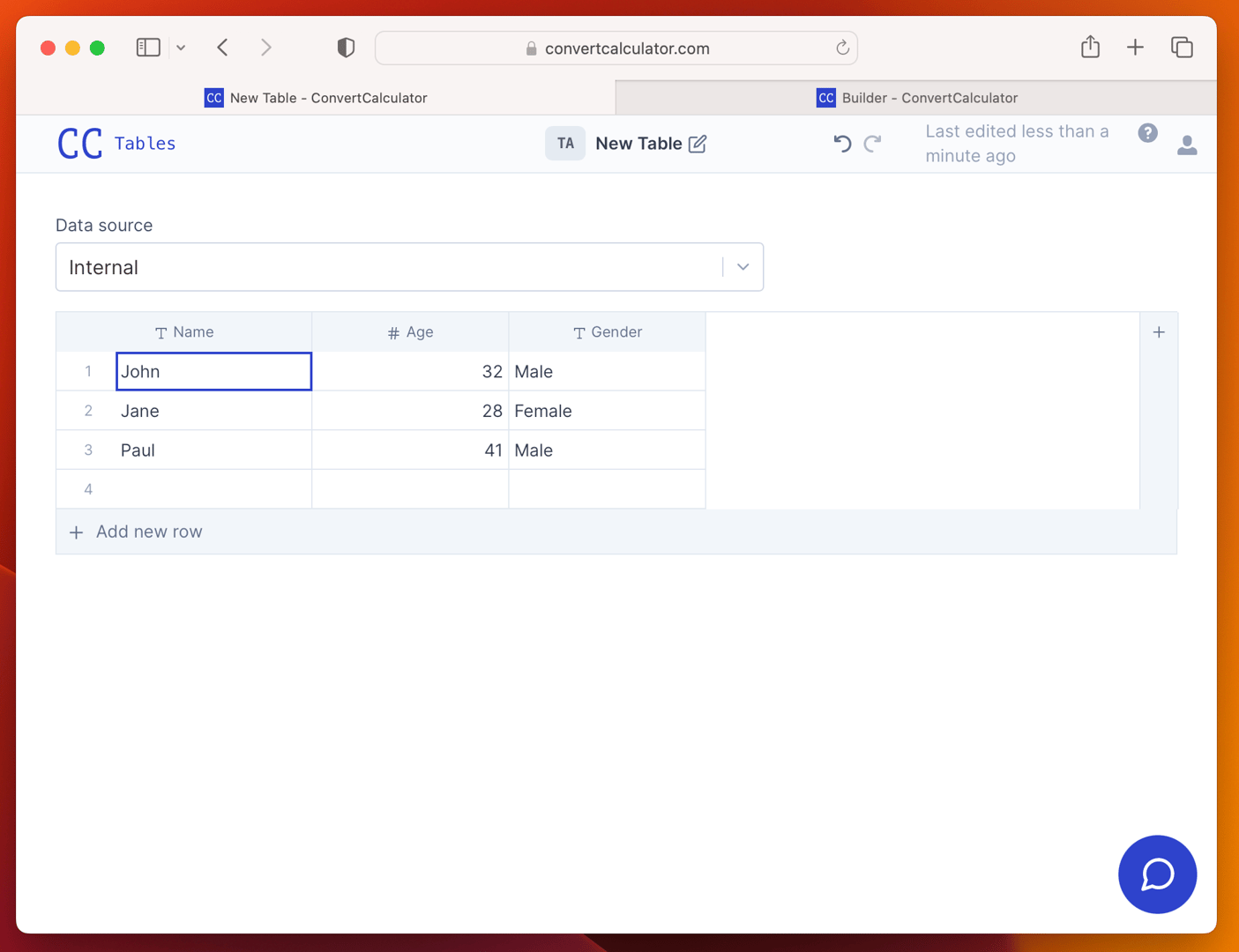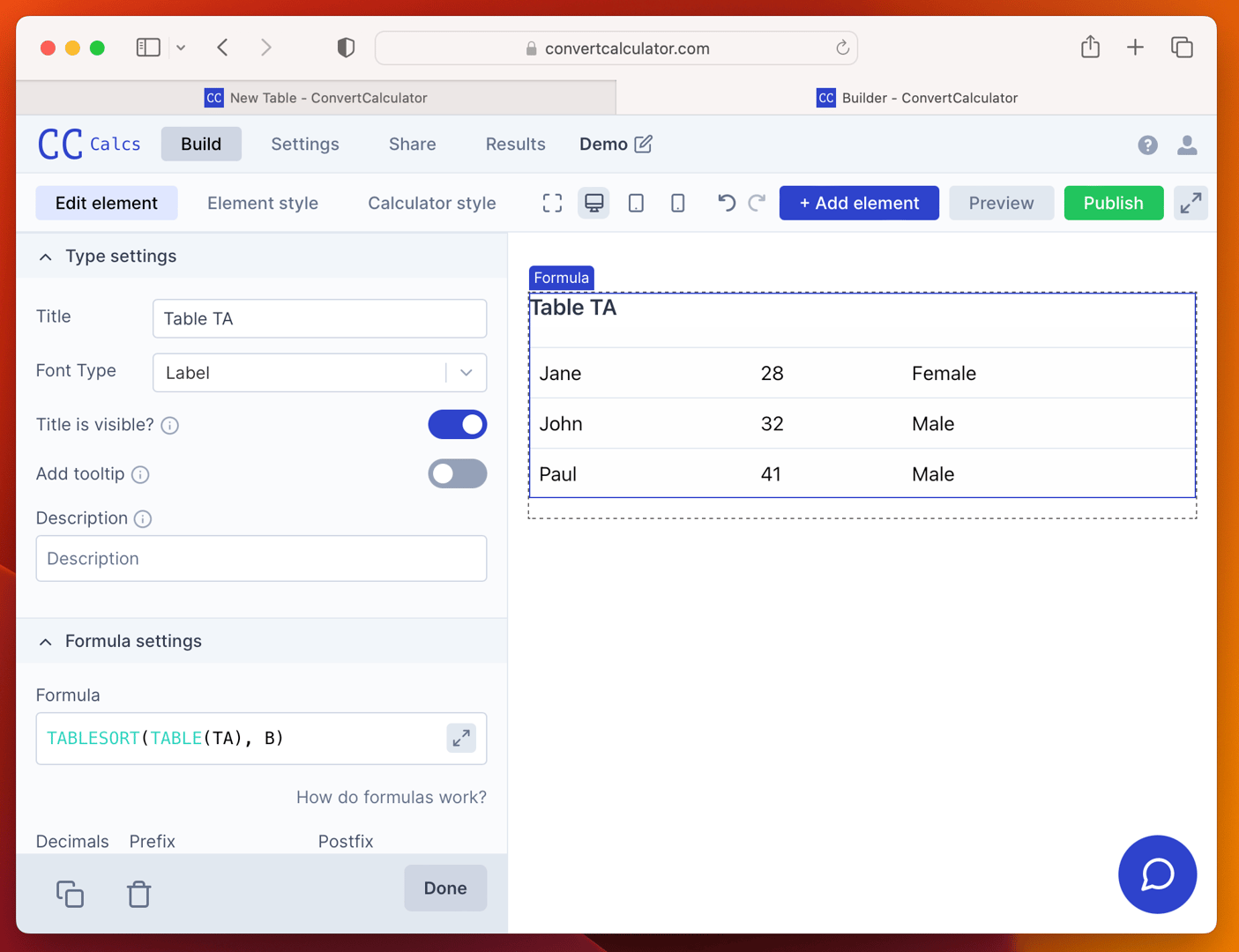# TABLESORT function

The TABLESORT function in Excel allows you to sort a Table or Datasheet based on a column

The TABLESORT function has the following syntax:
`FS`TABLESORT(reference, [sort_column], [sort_order], [by_col])``

Where:

• `reference` is the Table or Datasheet you want to sort.
• `sort_column` [optional] is the index of the column or row that you want to sort by. If `reference` is a Table or Datasheet, `sort_column` [optional] is the number of the column in the range. The default value is 0 (the first column)
• `sort_order` [optional] is a logical value that specifies the sort order. If `sort_order` is `1`, the `TABLESORT` function will sort the array in ascending order. If `sort_order` is `-1` , the `TABLESORT` function will sort the array in descending order. If you omit `sort_order`, the `TABLESORT` function will sort the array in ascending order.

For example, suppose you have a table TA of data as shown below:To sort Table TA by the Age column in ascending order, you could use the following TABLESORT function:

`TABLESORT(TABLE(TA), B)`

This function sorts TA by the second column (i.e., Age) in ascending order (i.e., smallest to largest). The result of the function would be the following table:## Related articles